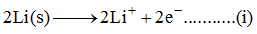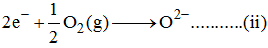Courses

# Balancing Of Redox Reactions - Redox Reactions Chemistry Notes | EduRev

## Chemistry : Balancing Of Redox Reactions - Redox Reactions Chemistry Notes | EduRev

The document Balancing Of Redox Reactions - Redox Reactions Chemistry Notes | EduRev is a part of the Chemistry Course Physical Chemistry.
All you need of Chemistry at this link: Chemistry

12. BALANCING OF REDOX REACTIONS

Oxidation-reduction or redox reactions involves electron transfer either between the atoms of same molecule or between the atoms of different molecules.  Redox reactions can take place in solution or in the gas phase, or they may be heterogeneous reactions (involving more than one phase ). Redox reactions take place between both inorganic and organic compounds.

A redox reaction is the sum of two half-reactions, one of which involves loss of electrons and the other gain of electrons. For example , reaction of Li with oxygen to form LiO2.

2Li (s) + ½ O2 ( g) → LiO2 (s)

In crystalline LiO2, lithium exists as Li+ ions and oxygen as oxide ions (O2-), so the two half-reactions are

Oxidation half–reaction:Reduction half–reaction:The two half-reactions on summing algebraically gives overall reactions. In reaction (i), metallic lithium loses electrons and becomes lithium ions. This process is known as oxidation and lithium is said to be oxidized. In reaction (ii), the oxygen gains electrons to become oxide ions and is said to be reduced. This process is known as reduction.

Thus, oxidation is defined as the loss of electrons and reduction is defined as the gain of electrons.   The species which gets oxidized and reduces others is called reducing agent or reductant and the species which gets reduced and oxidizes others is called oxidizing agent or oxidant .

12.1 THE OXIDATION STATE OF AN ELEMENT

To describe the changes that occur in oxidation-reduction reactions and to write the correctly balanced equations for such reactions, it is helpful to know the concept of the oxidation state ( or oxidation number ) of an atom.

For monoatomic ions , the oxidation state is simply the charge on the ion. For example, oxidation states of Na+, Mg2+ , Cl- and N3- are +1, +2, -1 and -3.respectively. For covalently bonded substances, the charge on an atom would be so small that it is impossible to calculate the exact charge on each atom in the molecule. Thus, oxidation state for covalently bonded molecule is defined as “ the charge an atom would possess if all the bonds associated with that atom are broken heterolytically considering them to be completely ionic.” For example, in NH3 there are three N-H bonds. If we consider all three N-H bonds to be ionic, then each H will possess a charge of +1 while N has the charge -3 ( because N is more electronegative than H ). In H2O2, there are two O-H bonds and one O-O bond as evident from the structural formula H-O-O-H . Assuming each O-H bond to be ionic, each H atom would possess +1 charge while each O possess -1 charge. The O-O bond is not ionic as the bond is between atoms of similar electronegativity.

It is not necessary to know the structure of a molecule in order to calculate the oxidation state of an atom in a molecule. The following set of bookkeeping rules are used to assign the oxidation states of atoms in ions or molecules.

• Each pure element has an oxidation state of zero. This is true whether the element is a monoatomic gas, a metallic solid or a polyatomic molecule . Thus, Fe(s) , N2 (g), P4(s)and S8(s) are  all in zero oxidation state.

• In monoatomic ions, the oxidation state of element is equal to the charge on the ion. Thus, oxidation state of all alkali metals is +1 and that of alkaline earth metals is +2 an all their compounds. In ionic solid K2S , the oxidation states of potassium and sulphur are +1 and -2. In AgCl the oxidation state of silver and chlorine are +1 and -1 .

• The oxidation state of hydrogen in any molecule in which it is combined with another element is -2, except in the peroxides, the superoxides and in OF2. The peroxides ( H2O2 ,Na2O, BaOetc) are compounds in which there is an O-O covalent bond and the peroxide ion is O22-. The oxidation state of oxygen in peroxides is -1. The superoxides (like KO2 , CsO2 etc. ) are ionic compounds involving the superoxide ion O2- . the oxidation state of oxygen is ( -1/2 ) in superoxide ion . In OF2 , the oxidation of oxygen is +2 since fluorine is more electronegative than oxygen.

• The oxidation state of all the halogens is -1 in all their compounds except where they are combined with the an element of higher electronegativity or in interhalogen compounds. Oxidation state of fluorine is always -1, since it is the most electronegative element.

• In covalent compounds not involving hydrogen or oxygen , the more electronegative element is assigned negative oxidation state while less electronegative element is assigned positive oxidation state.

• The algebraic sum of the oxidation numbers of all the atoms combined in a molecule or complex ion must equal the net charge on the molecule or ion.

Redox Reactions involve oxidation and reduction both. Oxidation means loss of electrons and reduction means gain of electrons. Thus, redox reactions involve electron transfer and the number of electrons lost are the same as the number of electrons gained during the reaction. This aspect of redox reaction can serve as the basis of pattern for balancing redox reactions. There are two common and useful methods to balance the redox reactions. These are

1. Oxidation number method

2. Ion-electron method

12.2  BALANCING REDOX REACTIONS BY OXIDATION STATE METHOD

For balancing a redox reaction by oxidation number method , follow the order of steps as listed :

•  For each redox reaction, deduce the oxidation state of the elements that are undergoing oxidation and reduction.

• Separate the reactants and products into two half-reactions involving the elements that change their oxidation state. Write the skeletal equations for each half-reactions .

• For each half-reaction, first balance the number of atoms of the element undergoing change in oxidation state .

• Now find the total change in oxidation number by determining the change per atom and multiplying it by the total number of atoms that undergoes change. Also, decide whether electrons are lost or gained. An increase in oxidation state is loss of electrons and a decrease in oxidation state is gain of electrons.

• Add the electrons lost or gained to half-reactions. Lost electrons are placed on the product side and gained electrons are kept on the reactant side.

• Now add both the half-reactions after multiplying by suitable integers to make the number of electrons lost or gained same .

• Transfer the coefficients of each reactant and product to the main skeleton equations.

• If the coefficients developed are not correct, then change them by inspection. Such coefficient changes are required when an element from a compound goes in 2 different compounds, one with the same oxidation state and the other with different oxidation state.

• Count the charges on both sides of the equation and balance the charges in all the equations by adding requisite H+ or OH- to the required side. If the reaction occurs in acidic solution , use H+ and if it occurs in basic solution , use OH- . if the reaction occurs in neutral solution, use Hor OH- or any of the side needed. i.e. in a neutral solution , if negative charges are needed for balancing, use OH- and if positive charges are needed , use H+ .

• Balance the hydrogens and oxygens by adding the appropriate number of H2O molecules on the required sides.

12.3 BALANCING THE REDOX REACTIONS BY ION-ELECTRON METHOD

This method of balancing redox reactions involve following steps:

• For each redox reaction, deduce the oxidation state of the elements that are undergoing oxidation and reduction.

• Separate the reactants and products into two half-reactions involving the elements that change their oxidation state. Write the skeletal equations for each half-reactions.

• Balance each half-reaction separately involving given steps

1. First balance the atoms of the element undergoing oxidation or reduction.

2. Then balance atoms the elements other than hydrogen and oxygen.

3. For balancing oxygen atoms in acidic or neutral medium, add suitable number of H2O molecules to the side deficient in O while in alkaline medium, add equal number of H2O molecules as the excess of O on the side having excess of O atoms and add double the number of OH- ions on the opposite side of the equation.

4. In order to balance the hydrogen atoms in acidic or neutral medium, add required number of Hto the side deficient in H while in alkaline medium , add equal number of OH- ions as the excess number of H atoms on the side having excess H and equal number of H2O molecules on the opposite side of the equation.

•  Multiply each half-raction by suitable integer to make the number of electrons lost and gained same and add both the half-equations to get a completely balanced reaction.

Offer running on EduRev: Apply code STAYHOME200 to get INR 200 off on our premium plan EduRev Infinity!

## Physical Chemistry

84 videos|106 docs|31 tests

,

,

,

,

,

,

,

,

,

,

,

,

,

,

,

,

,

,

,

,

,

;